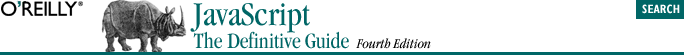home | O'Reilly's CD bookshelfs | FreeBSD | Linux | Cisco | Cisco Exam## 5.9. Assignment Operators

`i = 0`

While you might not normally think of such a line of JavaScript as an expression that can be evaluated, it is in fact an expression and, technically speaking, = is an operator.

The = operator expects its lefthand operand to be either a variable, the element of an array, or a property of an object. It expects its righthand operand to be an arbitrary value of any type. The value of an assignment expression is the value of the righthand operand. As a side effect, the = operator assigns the value on the right to the variable, element, or property on the left, so that future uses of the variable, element, or property refer to the value.

Because = is defined as an operator, you can include it in more complex expressions. For example, you can assign and test a value in the same expression with code like this:

`(a = b) == 0`

If you do this, be sure you are clear on the difference between the = and == operators!

The assignment operator has right-to-left associativity, which means that when multiple assignment operators appear in an expression, they are evaluated from right to left. Thus, you can write code like this to assign a single value to multiple variables:

`i = j = k = 0; `

Remember that each assignment expression has a value that is the value of the righthand side. So in the above code, the value of the first assignment (the rightmost one) becomes the righthand side for the second assignment (the middle one), and this value becomes the righthand side for the last (leftmost) assignment.